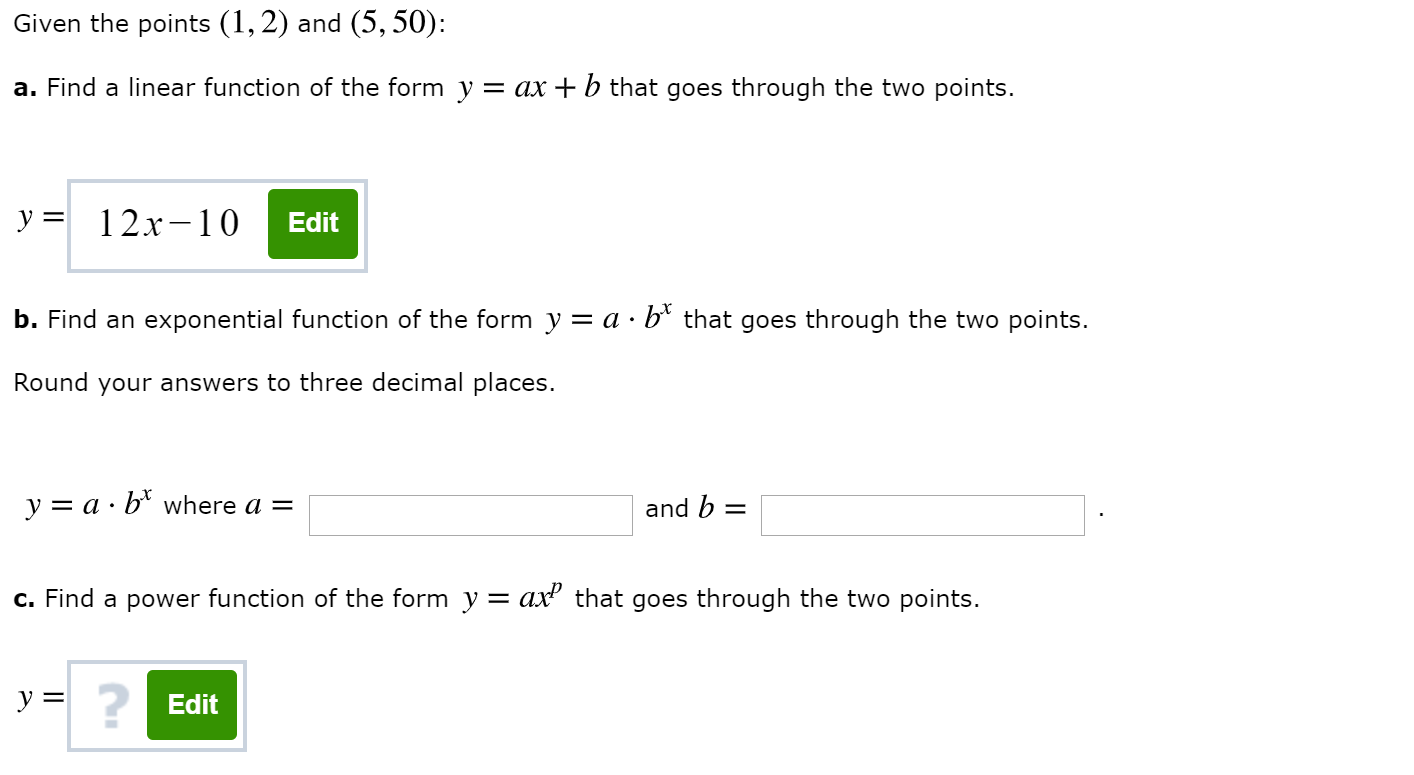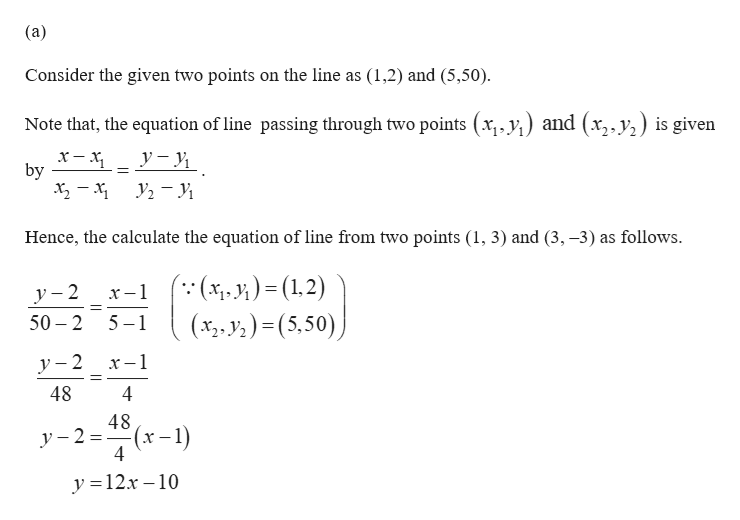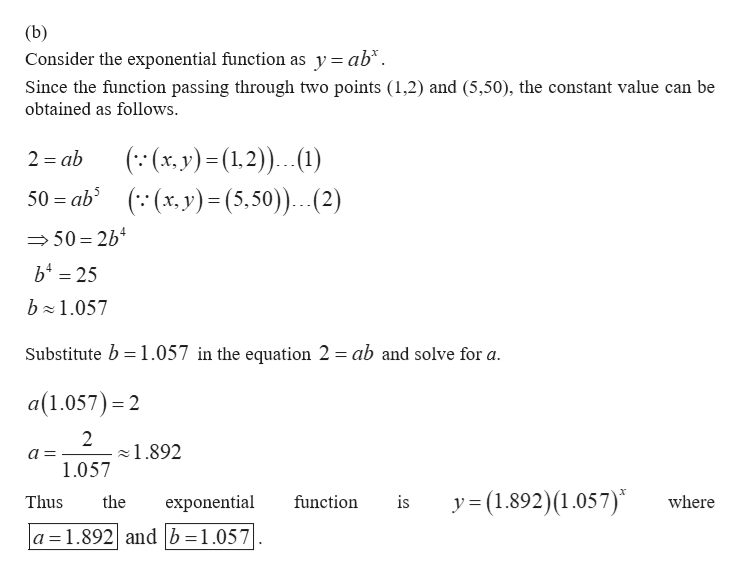# Given the points (1,2) and (5, 50):a. Find a linear function of the form y = ax +b that goes through the two points.y 12x-10Editb. Find an exponential function of the form y = a - b* that goes through the two points.Round your answers to three decimal places.y a b*where a =and bc. Find a power function of the form y = ax2 that goes through the two points.y Edit

Question
3 views

Question in Photohelp_outlineImage TranscriptioncloseGiven the points (1,2) and (5, 50): a. Find a linear function of the form y = ax +b that goes through the two points. y 12x-10 Edit b. Find an exponential function of the form y = a - b* that goes through the two points. Round your answers to three decimal places. y a b*where a = and b c. Find a power function of the form y = ax2 that goes through the two points. y Edit fullscreen
check_circle

Step 1help_outlineImage Transcriptionclose(a) Consider the given two points on the line as (1,2) and (5,50). Note that, the equation of line passing through two points (x,,y) and (x,,y) is given by Hence, the calculate the equation of line from two points (1, 3) and (3, -3) as follows ( (x, 1;) = (1,2) (x,.y2)(5,50) y-2 50 2 x-1 5 1 -1 y-2 48 4 48 y-2 4 (x-1) y 12x-10 fullscreen
Step 2help_outlineImage Transcriptionclose(b) Consider the exponential function as y = ab*. Since the function passing through two points (1,2) and (5,50), the constant value can be obtained as follows ((x,y)(1.2) 1) 50 ab ((x, y)= (5,50)...(2) 2 ab 50 2b4 b 25 b 1.057 2 Substitute b 1.057 in the equation 2 ab and solve for a a(1.057) 2 2 1.892 = 1057 y =(1.892)(1.057) Thus the exponential function is where a 1.892 and b=1.057 fullscreen

### Want to see the full answer?

See Solution

#### Want to see this answer and more?

Solutions are written by subject experts who are available 24/7. Questions are typically answered within 1 hour.*

See Solution
*Response times may vary by subject and question.
Tagged in

### Other